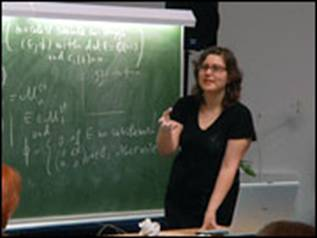• A
• A
• A
• АБВ
• АБВ
• АБВ
• А
• А
• А
• А
• А
Обычная версия сайта

Ruxandra Moraru

Ruxandra Moraru (Waterloo) посетила Лабораторию алгебраической геометрии в марте 2013 года. Она приняла участие в совместных исследованиях и сотрудничестве по теме "Голоморфные пучки на НКТ многообразиях", а также дважды выступила на еженедельном семинаре Лаборатории:

5 марта 2013 года с докладом "The geometry of essentially saturated spaces" и 15 марта 2013 года с докладом "Generalized holomorphic vector bundles".

The geometry of essentially saturated spaces

Abstract: A compact complex space X is called {\em essentially saturated} if and only if the components of the Douady space of each Cartesian power Xn are compact; these spaces are of special interest in geometric model theory because they are universal domains for their first-order theories. Examples of essentially saturated spaces are given by spaces of Fujiki class \mathcal{C} since the components of the Douady space of such spaces are always compact and the Cartesian product of two spaces of class \mathcal{C} is again of class \mathcal{C}. The class \mathcal{S} of essentially saturated spaces is thus a natural extension of the Fujiki class \mathcal{C}, and an interesting problem is to understand how these two classes differ. It is important to point out that not all compact complex spaces are essentially saturated; for example Hopf surfaces are not. Furthermore, one can show that there exist spaces of class \mathcal{S} that are not of class \mathcal{C}. In this talk, I will give examples of essentially saturated spaces that are not of Fujiki class \mathcal{C}\$and discuss some open questions.

This is joint work with Rahim Moosa and Matei Toma.

Generalized holomorphic vector bundles

Abstract: Generalized complex geometry is a hybrid of complex and symplectic geometry, introduced by Hitchin in 2002, which provides the proper framework for many questions arising in complex/Poisson geometry and in physics. Generalized holomorphic bundles are the analogues of holomorphic vector bundles in the generalized geometry setting. For some generalized complex structures, these bundles correspond to co-Higgs bundles, flat bundles or Poisson modules. In this talk, I will recall the notion of generalized complex structure and give an overview of what is known about generalized holomorphic bundles. I will also describe theirmoduli spaces in some specific examples.Нашли опечатку?
Выделите её, нажмите Ctrl+Enter и отправьте нам уведомление. Спасибо за участие!
Сервис предназначен только для отправки сообщений об орфографических и пунктуационных ошибках.